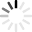Loading VESLUse the slider to multiply the basal friction coefficient α at the ice/bed interface (click INFO for a definition) by a scalar ranging from 5% to 200%.

This simulates a modification of the lubrication under the ice sheet from events such as increased precipitation, runoff, etc.

V and ΔV are the velocity and velocity change after friction scaling at the surface of the Greenland Ice Sheet, in meters per year.

%

## Info

### Introduction

This simulation of Greenland is the result of work carried out by the ISSM team for the SeaRISE experiments (Bindschadler et al., 2013, Nowicki et al. I, 2013, Nowicki et al. II, 2013) in which modeling teams from around the world compared their simulations against one another. One goal among others was to understand the impact of lubrication/friction at the ice/bedrock interface, and how the ice would speed-up if the friction coefficient α was reduced by a factor of two. Here, you will be able to replicate this experiment and play with a global reduction of Greenland friction coefficient up to 5% of its 2010 value.

### Datasets Used for the Simulation

• Surface elevation from Bamber et al., 2001.
• Bedrock elevation from Bamber et al., 2001.
• Surface velocities from Joughin et al., 2010.
• Basal heat flux from Shapiro and Ritzwoller, 2004.
• Other datasets found at the SeaRISE webpage.

### Model Settings

• Initial basal friction coefficient inverted from surface velocities (Joughin et al., 2010).
• Perturbation of the basal friction coefficient (range 5% to 200% of the original value).
• Steady-State simulation of surface velocity.
• Stress-Balance using the Shelfy Stream Approximation (MacAyeal et al., 1993).
• Basal stress at the ice/bed interface: τb = α2 · Neff · ub where α is the basal drag coefficient, Neff the effective water pressure and ub the ice/bed interface friction velocity.

### How to Run the Simulation

To start the simulation, scale the entire friction coefficient α by a scalar ranging from 5 to 200% using the slider, then click the "RUN" button. Results (ice surface velocity) will be computed by the JPL ISSM server, downloaded, and displayed.

### Feedback

If you have any questions or feedback, please send us an email.